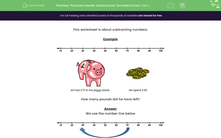# Practise Harder Subtraction: Numbers from 1 to 100

In this worksheet, students subtract numbers to find how much is left.Key stage:  KS 2

Curriculum topic:   Number: Addition and Subtraction

Curriculum subtopic:   Use Written Addition/Subtraction to Three Digits

Popular topics:   Subtraction worksheets

Difficulty level:#### Worksheet Overview

This worksheet is about subtracting numbers.

ExampleAli has £71 in his piggy bank. He spent £42.

How many pounds did he have left?

We use the number line belowWe start at 71 and go back 42 to get to 29.

When you go back 42, you can go back 1, then 10, then 10, then 10, then 10, then 1

71 - 42= 29

Ali has 29 pounds left.

### What is EdPlace?

We're your National Curriculum aligned online education content provider helping each child succeed in English, maths and science from year 1 to GCSE. With an EdPlace account you’ll be able to track and measure progress, helping each child achieve their best. We build confidence and attainment by personalising each child’s learning at a level that suits them.

Get started